# Aussem

18 Oct 2013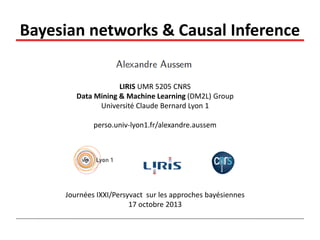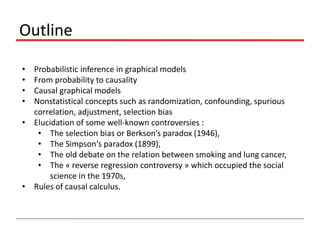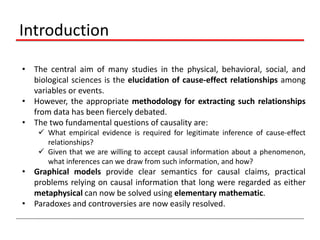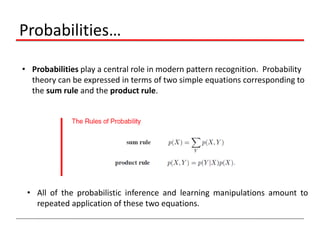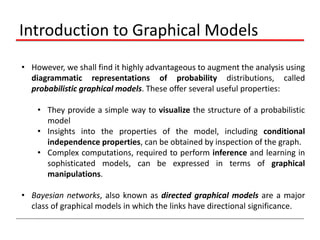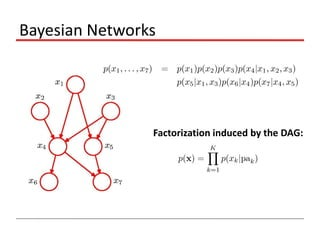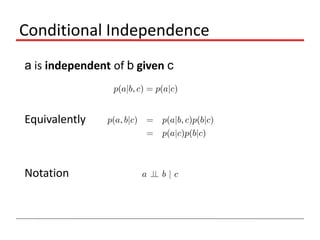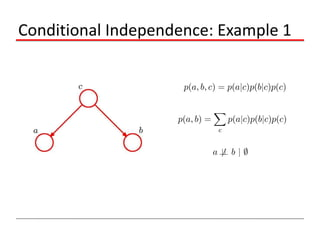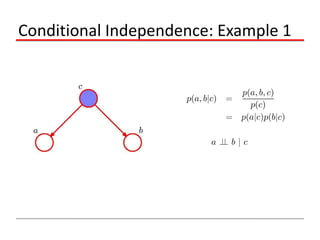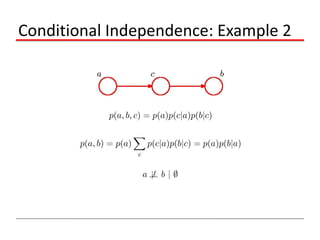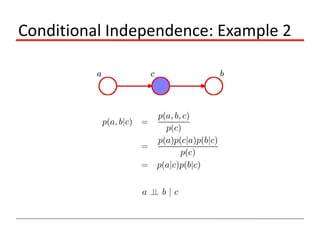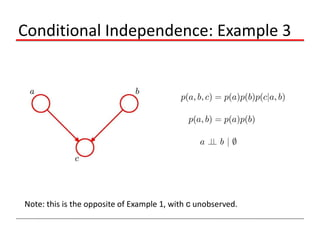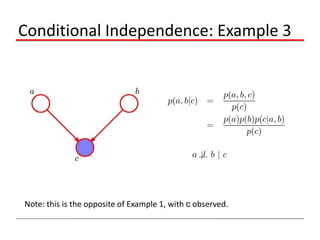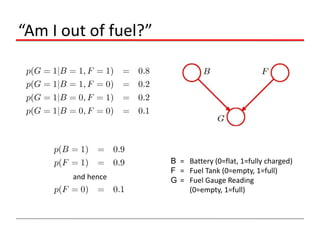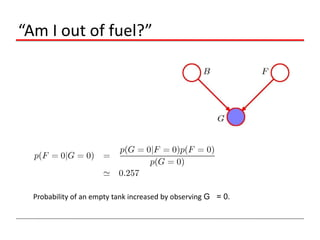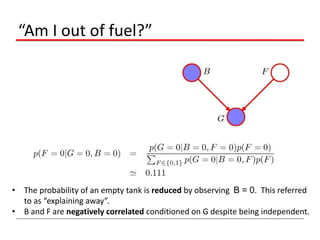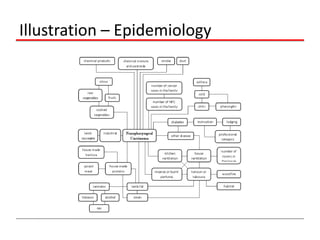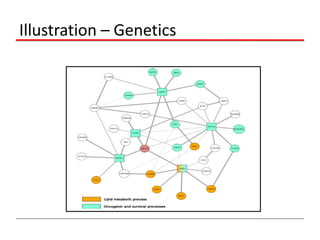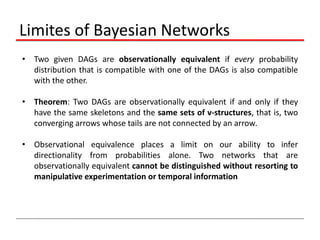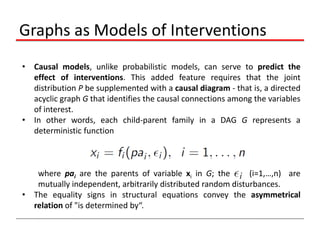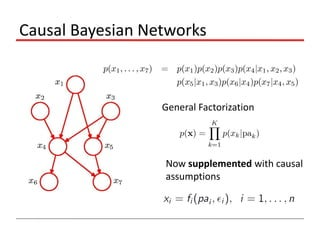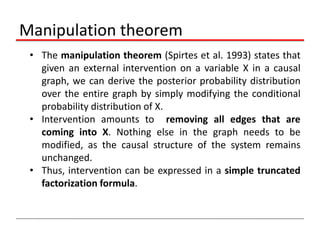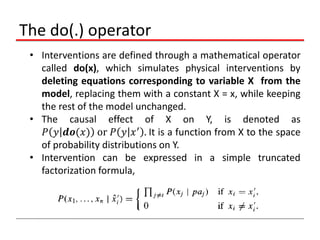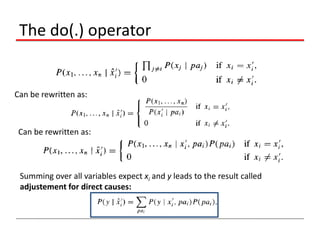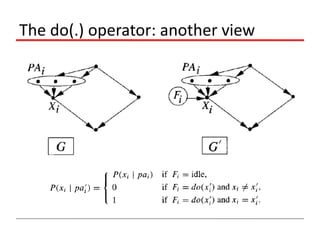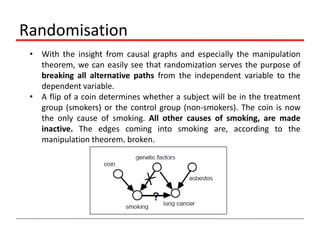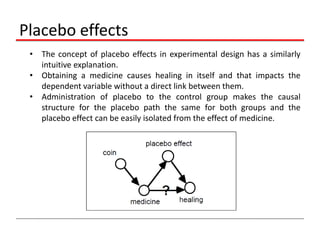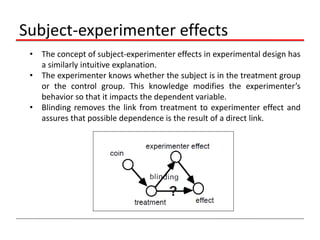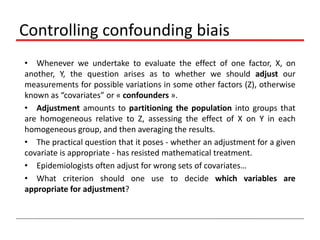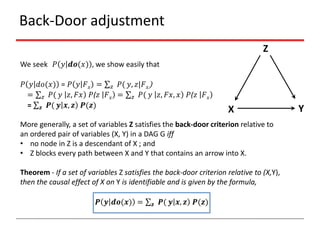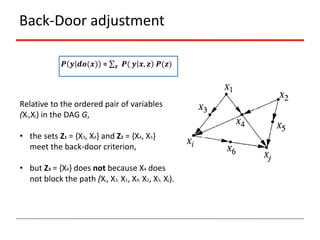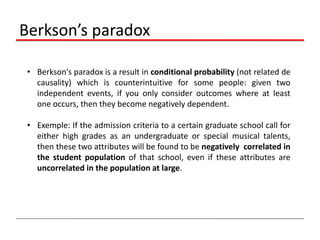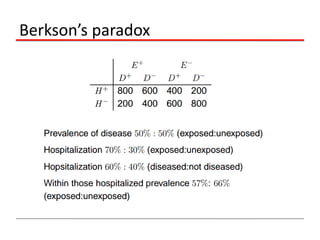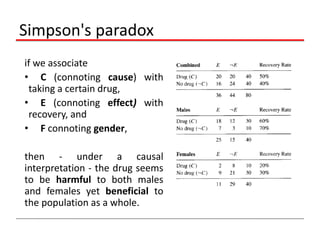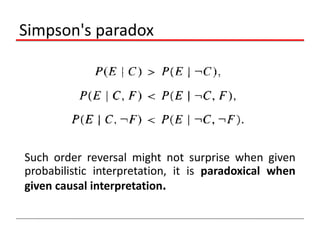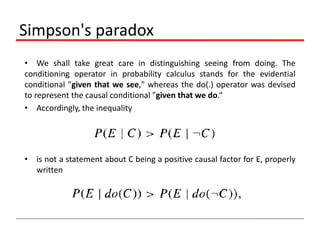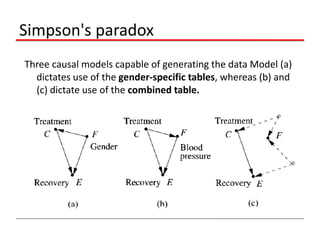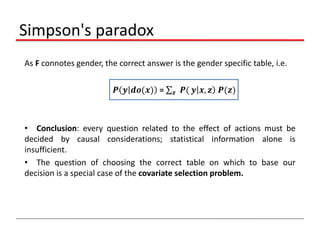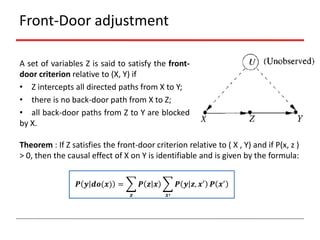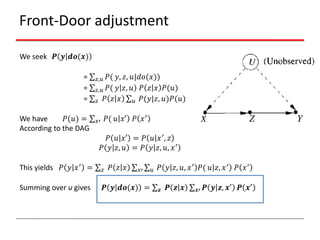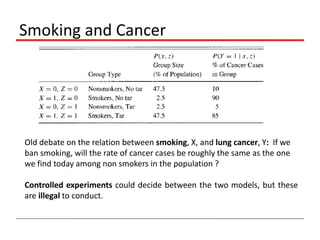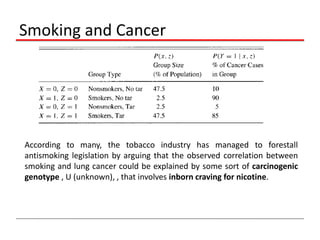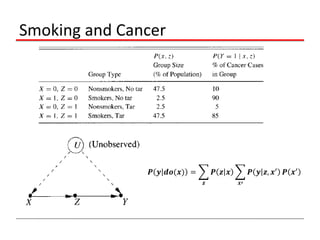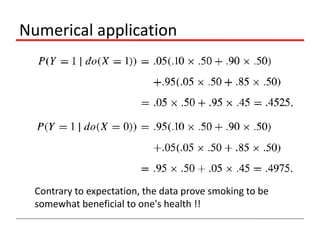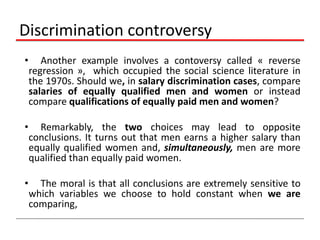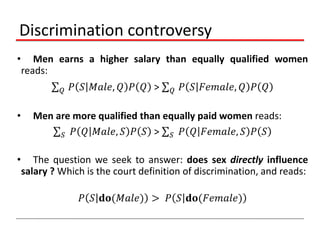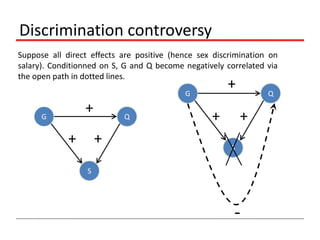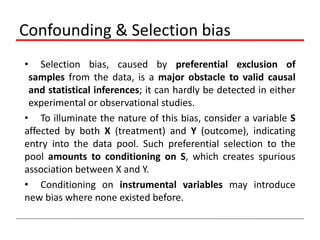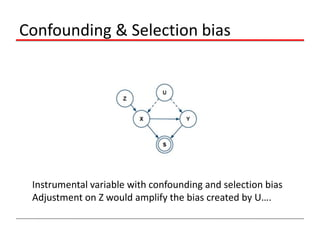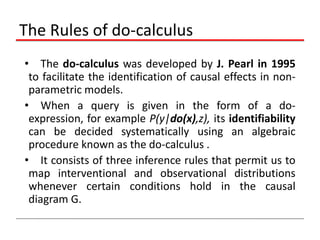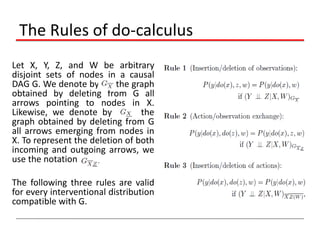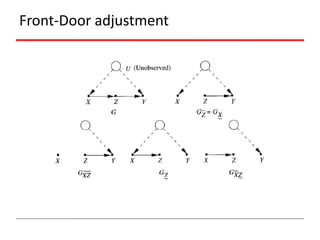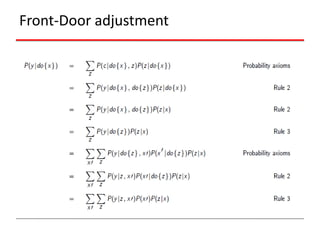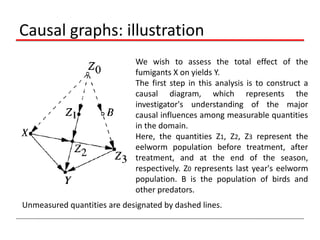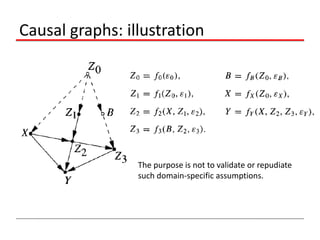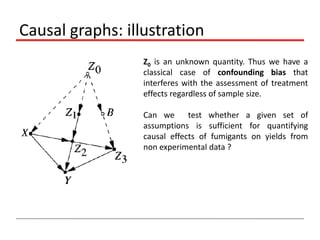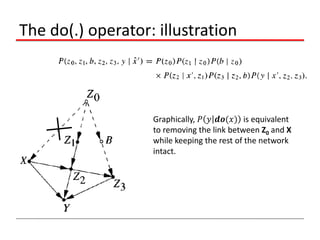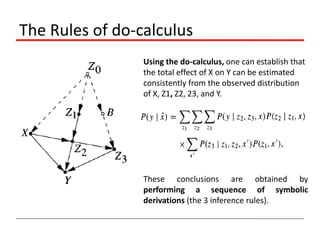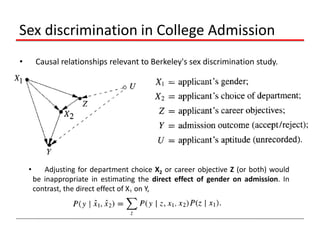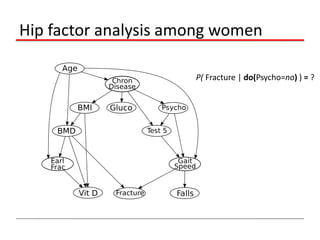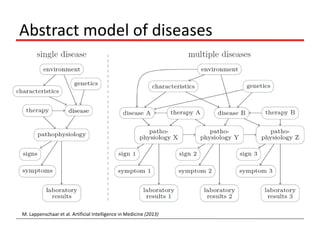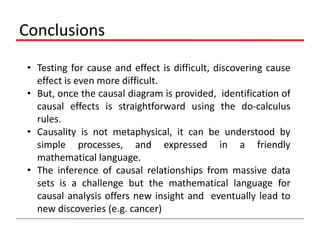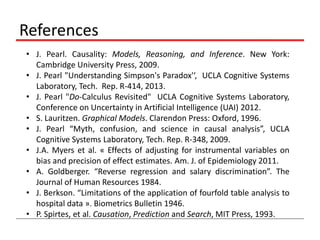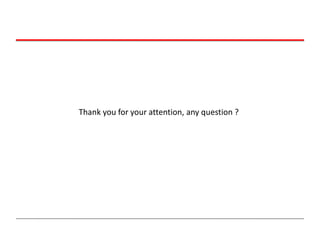1 sur 64

### Aussem

• 1. Bayesian networks & Causal Inference LIRIS UMR 5205 CNRS Data Mining & Machine Learning (DM2L) Group Université Claude Bernard Lyon 1 perso.univ-lyon1.fr/alexandre.aussem Journées IXXI/Persyvact sur les approches bayésiennes 17 octobre 2013
• 2. Outline • • • • Probabilistic inference in graphical models From probability to causality Causal graphical models Nonstatistical concepts such as randomization, confounding, spurious correlation, adjustment, selection bias • Elucidation of some well-known controversies : • The selection bias or Berkson’s paradox (1946), • The Simpson's paradox (1899), • The old debate on the relation between smoking and lung cancer, • The « reverse regression controversy » which occupied the social science in the 1970s, • Rules of causal calculus.
• 3. Introduction • The central aim of many studies in the physical, behavioral, social, and biological sciences is the elucidation of cause-effect relationships among variables or events. • However, the appropriate methodology for extracting such relationships from data has been fiercely debated. • The two fundamental questions of causality are:  What empirical evidence is required for legitimate inference of cause-effect relationships?  Given that we are willing to accept causal information about a phenomenon, what inferences can we draw from such information, and how? • Graphical models provide clear semantics for causal claims, practical problems relying on causal information that long were regarded as either metaphysical can now be solved using elementary mathematic. • Paradoxes and controversies are now easily resolved.
• 4. Probabilities… • Probabilities play a central role in modern pattern recognition. Probability theory can be expressed in terms of two simple equations corresponding to the sum rule and the product rule. • All of the probabilistic inference and learning manipulations amount to repeated application of these two equations.
• 5. Introduction to Graphical Models • However, we shall find it highly advantageous to augment the analysis using diagrammatic representations of probability distributions, called probabilistic graphical models. These offer several useful properties: • They provide a simple way to visualize the structure of a probabilistic model • Insights into the properties of the model, including conditional independence properties, can be obtained by inspection of the graph. • Complex computations, required to perform inference and learning in sophisticated models, can be expressed in terms of graphical manipulations. • Bayesian networks, also known as directed graphical models are a major class of graphical models in which the links have directional significance.
• 7. Conditional Independence a is independent of b given c Equivalently Notation
• 12. Conditional Independence: Example 3 Note: this is the opposite of Example 1, with c unobserved.
• 13. Conditional Independence: Example 3 Note: this is the opposite of Example 1, with c observed.
• 14. “Am I out of fuel?” and hence B = Battery (0=flat, 1=fully charged) F = Fuel Tank (0=empty, 1=full) G = Fuel Gauge Reading (0=empty, 1=full)
• 15. “Am I out of fuel?” Probability of an empty tank increased by observing G = 0.
• 16. “Am I out of fuel?” • The probability of an empty tank is reduced by observing B = 0. This referred to as “explaining away”. • B and F are negatively correlated conditioned on G despite being independent.
• 19. Limites of Bayesian Networks • Two given DAGs are observationally equivalent if every probability distribution that is compatible with one of the DAGs is also compatible with the other. • Theorem: Two DAGs are observationally equivalent if and only if they have the same skeletons and the same sets of v-structures, that is, two converging arrows whose tails are not connected by an arrow. • Observational equivalence places a limit on our ability to infer directionality from probabilities alone. Two networks that are observationally equivalent cannot be distinguished without resorting to manipulative experimentation or temporal information
• 20. Graphs as Models of Interventions • Causal models, unlike probabilistic models, can serve to predict the effect of interventions. This added feature requires that the joint distribution P be supplemented with a causal diagram - that is, a directed acyclic graph G that identifies the causal connections among the variables of interest. • In other words, each child-parent family in a DAG G represents a deterministic function where pai are the parents of variable xi in G; the (i=1,…,n) are mutually independent, arbitrarily distributed random disturbances. • The equality signs in structural equations convey the asymmetrical relation of "is determined by“.
• 21. Causal Bayesian Networks General Factorization Now supplemented with causal assumptions
• 22. Manipulation theorem • The manipulation theorem (Spirtes et al. 1993) states that given an external intervention on a variable X in a causal graph, we can derive the posterior probability distribution over the entire graph by simply modifying the conditional probability distribution of X. • Intervention amounts to removing all edges that are coming into X. Nothing else in the graph needs to be modified, as the causal structure of the system remains unchanged. • Thus, intervention can be expressed in a simple truncated factorization formula.
• 23. The do(.) operator • Interventions are defined through a mathematical operator called do(x), which simulates physical interventions by deleting equations corresponding to variable X from the model, replacing them with a constant X = x, while keeping the rest of the model unchanged. • The causal effect of X on Y, is denoted as 𝑃 𝑦 𝒅𝒐(𝑥) or 𝑃 𝑦 𝑥 ′ . It is a function from X to the space of probability distributions on Y. • Intervention can be expressed in a simple truncated factorization formula,
• 24. The do(.) operator Can be rewritten as: Can be rewritten as: Summing over all variables expect xi and y leads to the result called adjustement for direct causes:
• 25. The do(.) operator: another view Graphically, is equivalent to removing the ling between Z0 and X while keeping the rest of the network intact.
• 26. Randomisation • With the insight from causal graphs and especially the manipulation theorem, we can easily see that randomization serves the purpose of breaking all alternative paths from the independent variable to the dependent variable. • A flip of a coin determines whether a subject will be in the treatment group (smokers) or the control group (non-smokers). The coin is now the only cause of smoking. All other causes of smoking, are made inactive. The edges coming into smoking are, according to the manipulation theorem, broken.
• 27. Placebo effects • The concept of placebo effects in experimental design has a similarly intuitive explanation. • Obtaining a medicine causes healing in itself and that impacts the dependent variable without a direct link between them. • Administration of placebo to the control group makes the causal structure for the placebo path the same for both groups and the placebo effect can be easily isolated from the effect of medicine.
• 28. Subject-experimenter effects • The concept of subject-experimenter effects in experimental design has a similarly intuitive explanation. • The experimenter knows whether the subject is in the treatment group or the control group. This knowledge modifies the experimenter’s behavior so that it impacts the dependent variable. • Blinding removes the link from treatment to experimenter effect and assures that possible dependence is the result of a direct link.
• 29. Controlling confounding biais • Whenever we undertake to evaluate the effect of one factor, X, on another, Y, the question arises as to whether we should adjust our measurements for possible variations in some other factors (Z), otherwise known as “covariates” or « confounders ». • Adjustment amounts to partitioning the population into groups that are homogeneous relative to Z, assessing the effect of X on Y in each homogeneous group, and then averaging the results. • The practical question that it poses - whether an adjustment for a given covariate is appropriate - has resisted mathematical treatment. • Epidemiologists often adjust for wrong sets of covariates… • What criterion should one use to decide which variables are appropriate for adjustment?
• 30. Back-Door adjustment Z We seek 𝑃 𝑦 𝒅𝒐(𝑥) , we show easily that 𝑃 𝑦 𝑑𝑜(𝑥) = 𝑃 𝑦 𝐹 𝑥 = 𝑧 𝑃( 𝑦, 𝑧|𝐹 𝑥 ) = 𝑧 𝑃( 𝑦 𝑧, 𝐹𝑥 𝑃(𝑧 𝐹 𝑥 = 𝑧 𝑃( 𝑦 𝑧, 𝐹𝑥, 𝑥 𝑃(𝑧 𝐹 𝑥 = 𝒛 𝑷( 𝒚 𝒙, 𝒛 𝑷(𝒛) X More generally, a set of variables Z satisfies the back-door criterion relative to an ordered pair of variables (X, Y) in a DAG G iff • no node in Z is a descendant of X ; and • Z blocks every path between X and Y that contains an arrow into X. Theorem - If a set of variables Z satisfies the back-door criterion relative to (X,Y), then the causal effect of X on Y is identifiable and is given by the formula, 𝑷 𝒚 𝒅𝒐(𝒙) = 𝒛 𝑷( 𝒚 𝒙, 𝒛 𝑷(𝒛) Y
• 31. Back-Door adjustment 𝑷 𝒚 𝒅𝒐(𝒙) = 𝒛 𝑷( 𝒚 𝒙, 𝒛 𝑷(𝒛) Relative to the ordered pair of variables (Xi,Xj) in the DAG G, • the sets Z1 = {X3, X4} and Z2 = {X4, X5} meet the back-door criterion, • but Z3 = {X4} does not because X4 does not block the path (Xi, X3, X1, X4, X2, X5, Xj).
• 32. Berkson’s paradox • Berkson's paradox is a result in conditional probability (not related de causality) which is counterintuitive for some people: given two independent events, if you only consider outcomes where at least one occurs, then they become negatively dependent. • Exemple: If the admission criteria to a certain graduate school call for either high grades as an undergraduate or special musical talents, then these two attributes will be found to be negatively correlated in the student population of that school, even if these attributes are uncorrelated in the population at large.
• 34. Simpson's paradox if we associate • C (connoting cause) with taking a certain drug, • E (connoting effect) with recovery, and • F connoting gender, then - under a causal interpretation - the drug seems to be harmful to both males and females yet beneficial to the population as a whole.
• 35. Simpson's paradox Such order reversal might not surprise when given probabilistic interpretation, it is paradoxical when given causal interpretation.
• 36. Simpson's paradox • We shall take great care in distinguishing seeing from doing. The conditioning operator in probability calculus stands for the evidential conditional "given that we see," whereas the do(.) operator was devised to represent the causal conditional "given that we do.“ • Accordingly, the inequality • is not a statement about C being a positive causal factor for E, properly written
• 37. Simpson's paradox Three causal models capable of generating the data Model (a) dictates use of the gender-specific tables, whereas (b) and (c) dictate use of the combined table.
• 38. Simpson's paradox As F connotes gender, the correct answer is the gender specific table, i.e. 𝑷 𝒚 𝒅𝒐(𝒙) = 𝒛 𝑷( 𝒚 𝒙, 𝒛 𝑷(𝒛) • Conclusion: every question related to the effect of actions must be decided by causal considerations; statistical information alone is insufficient. • The question of choosing the correct table on which to base our decision is a special case of the covariate selection problem.
• 39. Front-Door adjustment A set of variables Z is said to satisfy the frontdoor criterion relative to (X, Y) if • Z intercepts all directed paths from X to Y; • there is no back-door path from X to Z; • all back-door paths from Z to Y are blocked by X. Theorem : If Z satisfies the front-door criterion relative to ( X , Y) and if P(x, z ) > 0, then the causal effect of X on Y is identifiable and is given by the formula: 𝑷 𝒚 𝒅𝒐(𝒙) = 𝑷 𝒚 𝒛, 𝒙′ 𝑷 𝒙′ 𝑷 𝒛 𝒙 𝒛 𝒙′
• 40. Front-Door adjustment We seek 𝑷 𝒚 𝒅𝒐(𝒙) = = = We have 𝑃(𝑢) = According to the DAG This yields 𝑃 𝑦 𝑥 ′ = Summing over u gives 𝑃( 𝑦, 𝑧, 𝑢|𝑑𝑜(𝑥)) 𝑧,𝑢 𝑃( 𝑦 𝑧, 𝑢 𝑃 𝑧 𝑥 𝑃(𝑢) 𝑧 𝑃 𝑧 𝑥 𝑢 𝑃(𝑦|𝑧, 𝑢)𝑃(𝑢) 𝑧,𝑢 𝑃( 𝑢 𝑥′ 𝑃 𝑥 ′ 𝑥′ 𝑃 𝑢 𝑥′ = 𝑃 𝑢 𝑥 ′ , 𝑧 𝑃 𝑦 𝑧, 𝑢 = 𝑃 𝑦 𝑧, 𝑢, 𝑥 ′ 𝑧 𝑃 𝑧 𝑥 𝑥′ 𝑷 𝒚 𝒅𝒐(𝒙) = 𝑢 𝑃 𝑦 𝑧, 𝑢, 𝑥 ′ 𝑃( 𝑢 𝑧, 𝑥 ′ 𝑃 𝑥 ′ 𝒛 𝑷 𝒛 𝒙 𝒙′ 𝑷 𝒚 𝒛, 𝒙′ 𝑷 𝒙′
• 41. Smoking and Cancer Old debate on the relation between smoking, X, and lung cancer, Y: If we ban smoking, will the rate of cancer cases be roughly the same as the one we find today among non smokers in the population ? Controlled experiments could decide between the two models, but these are illegal to conduct.
• 42. Smoking and Cancer According to many, the tobacco industry has managed to forestall antismoking legislation by arguing that the observed correlation between smoking and lung cancer could be explained by some sort of carcinogenic genotype , U (unknown), , that involves inborn craving for nicotine.
• 43. Smoking and Cancer 𝑷 𝒚 𝒅𝒐(𝒙) = 𝑷 𝒚 𝒛, 𝒙′ 𝑷 𝒙′ 𝑷 𝒛 𝒙 𝒛 𝒙′
• 44. Numerical application Contrary to expectation, the data prove smoking to be somewhat beneficial to one's health !!
• 45. Discrimination controversy • Another example involves a contoversy called « reverse regression », which occupied the social science literature in the 1970s. Should we, in salary discrimination cases, compare salaries of equally qualified men and women or instead compare qualifications of equally paid men and women? • Remarkably, the two choices may lead to opposite conclusions. It turns out that men earns a higher salary than equally qualified women and, simultaneously, men are more qualified than equally paid women. • The moral is that all conclusions are extremely sensitive to which variables we choose to hold constant when we are comparing,
• 46. Discrimination controversy • Men earns a higher salary than equally qualified women reads: 𝑄 • • 𝑃 𝑆 𝑀𝑎𝑙𝑒, 𝑄 𝑃 𝑄 > 𝑄 𝑃 𝑆 𝐹𝑒𝑚𝑎𝑙𝑒, 𝑄 𝑃 𝑄 Men are more qualified than equally paid women reads: 𝑆 𝑃 𝑄 𝑀𝑎𝑙𝑒, 𝑆 𝑃 𝑆 > 𝑆 𝑃 𝑄 𝐹𝑒𝑚𝑎𝑙𝑒, 𝑆 𝑃 𝑆 The question we seek to answer: does sex directly influence salary ? Which is the court definition of discrimination, and reads: 𝑃 𝑆 𝐝𝐨(𝑀𝑎𝑙𝑒) > 𝑃 𝑆 𝐝𝐨(𝐹𝑒𝑚𝑎𝑙𝑒)
• 47. Discrimination controversy Suppose all direct effects are positive (hence sex discrimination on salary). Conditionned on S, G and Q become negatively correlated via the open path in dotted lines. + G + G Q + + + Q + S S -
• 48. Confounding & Selection bias • Selection bias, caused by preferential exclusion of samples from the data, is a major obstacle to valid causal and statistical inferences; it can hardly be detected in either experimental or observational studies. • To illuminate the nature of this bias, consider a variable S affected by both X (treatment) and Y (outcome), indicating entry into the data pool. Such preferential selection to the pool amounts to conditioning on S, which creates spurious association between X and Y. • Conditioning on instrumental variables may introduce new bias where none existed before.
• 49. Confounding & Selection bias Instrumental variable with confounding and selection bias Adjustment on Z would amplify the bias created by U….
• 50. The Rules of do-calculus • The do-calculus was developed by J. Pearl in 1995 to facilitate the identification of causal effects in nonparametric models. • When a query is given in the form of a doexpression, for example P(y|do(x),z), its identifiability can be decided systematically using an algebraic procedure known as the do-calculus . • It consists of three inference rules that permit us to map interventional and observational distributions whenever certain conditions hold in the causal diagram G.
• 51. The Rules of do-calculus Let X, Y, Z, and W be arbitrary disjoint sets of nodes in a causal DAG G. We denote by the graph obtained by deleting from G all arrows pointing to nodes in X. Likewise, we denote by the graph obtained by deleting from G all arrows emerging from nodes in X. To represent the deletion of both incoming and outgoing arrows, we use the notation The following three rules are valid for every interventional distribution compatible with G.
• 54. Causal graphs: illustration We wish to assess the total effect of the fumigants X on yields Y. The first step in this analysis is to construct a causal diagram, which represents the investigator's understanding of the major causal influences among measurable quantities in the domain. Here, the quantities Z1, Z2, Z3 represent the eelworm population before treatment, after treatment, and at the end of the season, respectively. Z0 represents last year's eelworm population. B is the population of birds and other predators. Unmeasured quantities are designated by dashed lines.
• 55. Causal graphs: illustration The purpose is not to validate or repudiate such domain-specific assumptions.
• 56. Causal graphs: illustration Z0 is an unknown quantity. Thus we have a classical case of confounding bias that interferes with the assessment of treatment effects regardless of sample size. Can we test whether a given set of assumptions is sufficient for quantifying causal effects of fumigants on yields from non experimental data ?
• 57. The do(.) operator: illustration Graphically, 𝑃 𝑦 𝒅𝒐(𝑥) is equivalent to removing the link between Z0 and X while keeping the rest of the network intact.
• 58. The Rules of do-calculus Using the do-calculus, one can establish that the total effect of X on Y can be estimated consistently from the observed distribution of X, Z1, Z2, 23, and Y. It is given by the formula: These conclusions are obtained by performing a sequence of symbolic derivations (the 3 inference rules).
• 59. Sex discrimination in College Admission • Causal relationships relevant to Berkeley's sex discrimination study. • Adjusting for department choice X2 or career objective Z (or both) would be inappropriate in estimating the direct effect of gender on admission. In contrast, the direct effect of X1 on Y,
• 60. Hip factor analysis among women P( Fracture | do(Psycho=no) ) = ?
• 61. Abstract model of diseases M. Lappenschaar et al. Artificial Intelligence in Medicine (2013)
• 62. Conclusions • Testing for cause and effect is difficult, discovering cause effect is even more difficult. • But, once the causal diagram is provided, identification of causal effects is straightforward using the do-calculus rules. • Causality is not metaphysical, it can be understood by simple processes, and expressed in a friendly mathematical language. • The inference of causal relationships from massive data sets is a challenge but the mathematical language for causal analysis offers new insight and eventually lead to new discoveries (e.g. cancer)
• 63. References • J. Pearl. Causality: Models, Reasoning, and Inference. New York: Cambridge University Press, 2009. • J. Pearl "Understanding Simpson's Paradox'‘, UCLA Cognitive Systems Laboratory, Tech. Rep. R-414, 2013. • J. Pearl "Do-Calculus Revisited" UCLA Cognitive Systems Laboratory, Conference on Uncertainty in Artificial Intelligence (UAI) 2012. • S. Lauritzen. Graphical Models. Clarendon Press: Oxford, 1996. • J. Pearl “Myth, confusion, and science in causal analysis”, UCLA Cognitive Systems Laboratory, Tech. Rep. R-348, 2009. • J.A. Myers et al. « Effects of adjusting for instrumental variables on bias and precision of effect estimates. Am. J. of Epidemiology 2011. • A. Goldberger. “Reverse regression and salary discrimination”. The Journal of Human Resources 1984. • J. Berkson. “Limitations of the application of fourfold table analysis to hospital data ». Biometrics Bulletin 1946. • P. Spirtes, et al. Causation, Prediction and Search, MIT Press, 1993.
• 64. Thank you for your attention, any question ?